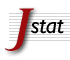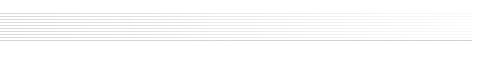| general | manuscript preparation | file and archive preparation | submission | | F.A.Q. | after submission | coauthors | the preprint web page | proof-reading and publication | | keyword list | copyright and license information | access to your article |

## Keyword List

 Note The keywords are organized in sections. The section name is highlighted in grey followed by the list of individual keywords belonging to that section.

 Group: JSTAT Keywords
Quantum statistical physics, condensed matter, integrable systems Classical statistical mechanics, equilibrium and non-equilibrium
• Algebraic structures of integrable models
• Anderson model
• Anyons and Fractional statistical models
• Bootstrap S-matrix
• Bose Einstein condensation
• Bosonisation
• Breaking integrability
• Classical integrability
• Cold Atoms
• Conformal field theory
• Connections between chaos and statistical physics
• Correlation functions
• Density matrix renormalisation group
• Dimers
• Dissipative systems
• Edge states
• Entanglement entropies
• Entanglement in extended quantum systems
• Entanglement in topological phase
• Form factors
• Fractional QHE
• Fractional Statistics
• Frustrated systems classical and quantum
• Gauge symmetry and gauge fields
• Generalized Gibbs Ensemble
• Graphene
• Hubbard and related model
• Integrable quantum field theory
• Integrable spin chains and vertex models
• Kondo effect
• Lieb-Liniger model
• Loop models and polymers
• Luttinger liquids
• Majorana fermion
• Matrix models
• Mesoscopic systems
• Non-linear Schroedinger equation
• Optical lattices
• Painlevé equations
• Quantum chaos
• Quantum computation
• Quantum criticality
• Quantum defects
• Quantum dimer and quantum loop models
• Quantum Disordered Systems
• Quantum Dissipative Systems
• Quantum dots
• Quantum fluids
• Quantum Frustrated systems
• Quantum gases
• Quantum integrability (Bethe Ansatz)
• Quantum Monte Carlo simulations
• Quantum Phase Transitions
• Quantum quenches
• Quantum Thermalization
• Quantum transport in one-dimension
• Quantum wires
• Quench Action
• Random Geometry
• Rigorous results in statistical mechanics
• Sigma models
• Solvable lattice models
• Spin chains, ladders and planes
• Spin liquids
• Stochastic Loewner evolution
• Superfluidity
• Symmetries of integrable models
• Tensor network simulations
• Thermalization
• Thermodynamic Bethe Ansatz
• Topological insulators
• Topological phases of matter
• Topology and combinatorics
• Absorbing states
• Active matter
• Avalanches
• Barkhausen noise
• Biological fluid dynamics
• Biopolymers
• Boltzmann equation
• Boundary layers
• Brownian motion
• Bubbles and drops
• Casimir effect
• Cellular automata
• Charged fluids
• Chemical kinetics
• Chemical vapor deposition
• Classical Monte Carlo simulations
• Classical phase transitions
• Cluster aggregation
• Coarse-graining
• Coarsening processes
• Colloids, bio-colloids and nano-colloids
• Combustion
• Compressible flow
• Computational fluid dynamics
• Correlation functions
• Critical exponents and amplitudes
• Current fluctuations
• Defects
• Dendritic growth
• Diffusion
• Diffusion-limited aggregation
• Discrete fluid models
• Driven diffusive systems
• Dynamical processes
• Elasticity
• Emulsions
• Exact results
• Exclusion processes
• Extreme value
• Films, foams and surfactants
• Finite-size scaling
• First Passage
• Fluctuating hydrodynamics
• Fluctuation phenomena
• Fluctuation theorems
• Fractal growth
• Fracture
• Free boundary problems
• Friction
• Gases dynamics
• Granular Material
• Growth processes
• Heat conduction
• Heteroepitaxy
• Interfaces in random media
• Irreversible aggregation phenomena
• Kinetic growth processes
• Kinetic Ising models
• Kinetic roughening
• Kinetic theory of gases and liquids
• Large deviations in non-equilibrium systems
• Lattice Boltzmann methods
• Liquid films
• Long-range interactions
• Macroscopic fluctation theory
• Metastable states
• Molecular beam epitaxy
• Molecular dynamics
• Non-equilibrium wetting
• Nucleation
• Numerical simulations
• Percolation problems
• Persistence
• Phase diagrams
• Plasticity
• Random/ordered microstructure
• Renewal Processes
• Renormalisation group
• Roughening transition
• Sampling algorithms, mixing
• Sandpile Models
• Self-affine roughness
• Self-organized criticality
• Series expansions
• Stationary states
• Stochastic particle dynamics
• Stochastic thermodynamics
• Structural correlations
• Surface diffusion
• Surface effects
• Surface migration
• Surface reconstructions
• Surface spectroscopy, STM etc.
• Traffic models
• Transport processes / heat transfer
• Transport properties
• Viscoelasticity
• Vortex matter
• Wetting
• Zero-range processes
Disordered systems, classical and quantum Interdisciplinary statistical mechanics
• Cavity and replica method
• Colloidal glasses
• Defects
• Diffusion in random media
• Disordered spin chains
• Dynamical heterogeneities
• Energy landscapes
• Ergodicity breaking
• Extreme value statistics
• Fracture
• Friction
• Gauge theories
• Glasses (colloidal, polymer, etc.)
• Glasses (structural)
• Heterogeneous materials
• Large deviation theory and Griffiths singularities
• Many body localization
• Memory effects
• Mode coupling theory
• Phase transitions into absorbing states
• Plasticity
• Polymers
• Quantum glasses
• Quantum transport
• Random matrix theory and extensions
• Random/ordered microstructures
• Slow relaxation, glassy dynamics, aging
• Spin glasses
• Transport properties
• Vortex matter
• Agent-based models
• Algorithmic game theory
• Analysis of algorithms
• Channel coding
• Clustering techniques
• Cognitive dynamical networks
• Communication, supply and information networks
• Critical phenomena of socio-economic systems
• Data mining
• Deep learning
• Dynamics of supply and production networks
• Error-correcting codes
• Evolutionary game theory
• Extreme value statistics
• Financial instruments and regulation
• Financial networks
• First Passage
• Game theory
• General equilibrium models
• Graphical Games
• Heuristics algorithms
• Inference in socio-economic system
• Inference of graphical models
• Information technology networks
• Large deviation
• Learning theory
• Machine Learning
• Market microstructure, market impact
• Mathematical economics
• Message-passing algorithms
• Models of financial markets
• Network dynamics
• Network reconstruction
• Nonlinear dynamics
• Online dynamics
• Optimization over networks
• Optimization under uncertainty
• Peer-to-peer networks
• Production networks
• Quantitative finance
• Quantum computation
• Quantum information
• Random graphs, networks
• Renewal Processes
• Risk measure and management
• Robust and stochastic optimisation
• Scaling in socio-economic systems
• Socio-economic networks
• Source coding
• Statistical inference
• Statistical Mechanics of Geophysical Systems
• Stochastic processes
• Stochastic search
• Systemic stability
• Traffic and crowd dynamics
• Traffic models
• Typical-case computational complexity
Biological modelling and information
• Active matter
• Active membranes
• Aggregation
• Biofilms
• Bioinformatics
• Biological fluid dynamics
• Biomolecules
• Biopolymers
• Cell-cell signaling
• Co-evolution
• Computational biology
• Computational neuroscience
• Data mining
• Deep learning
• Epidemic modelling
• Evolution models
• Evolutionary and comparative genomics
• Evolutionary processes
• Experiment design
• Gene expression and regulation
• Genetics
• Genomic and proteomic networks
• High-throughput screening
• Information processing
• Mechanical properties (DNA, RNA, membranes, bio-polymers)
• Metabolic control
• Metabolic networks
• Microfluidics
• Molecular motors
• Molecular networks
• Motif finding
• Multiple sequence alignment
• Neural code
• Neuromorphic models
• Neuronal networks
• Noise models
• Pattern formation
• Phylogeny
• Population dynamics
• Post-transcriptional regulation
• Protein folding
• Protein function and design
• Protein interaction networks
• Regulatory networks
• Self-propelled particles
• Sequence analysis
• Signal transduction
• Single cell genomics
• Single molecule
• Statistical Inference in biological systems
• Structures and conformations
• Synaptic plasticity
• Systems biology
• Systems neuroscience